Courses

# Test: Graph Based Algorithms- 2

## 20 Questions MCQ Test Algorithms | Test: Graph Based Algorithms- 2

Description
This mock test of Test: Graph Based Algorithms- 2 for Computer Science Engineering (CSE) helps you for every Computer Science Engineering (CSE) entrance exam. This contains 20 Multiple Choice Questions for Computer Science Engineering (CSE) Test: Graph Based Algorithms- 2 (mcq) to study with solutions a complete question bank. The solved questions answers in this Test: Graph Based Algorithms- 2 quiz give you a good mix of easy questions and tough questions. Computer Science Engineering (CSE) students definitely take this Test: Graph Based Algorithms- 2 exercise for a better result in the exam. You can find other Test: Graph Based Algorithms- 2 extra questions, long questions & short questions for Computer Science Engineering (CSE) on EduRev as well by searching above.
QUESTION: 1

### How many undirected graphs (not necessarily connected) can be constructed out of a given set V = {v1, v2, ... vn} of n vertices?

Solution:

There are total n*(n-1)/2 possible edges. For every edge, there are to possible options, either we pick it or don't pick. So total number of possible graphs is 2n(n-1)/2.

QUESTION: 2

### If the DFS finishing time f[u] > f[v] for two vertices u and v in a directed graph G, and u and v are in the same DFS tree in the DFS forest, then u is an ancestor of v in the depth first tree.

Solution:

In a graph with three nodes, r u and v, with edges (r; u) and (r; v), and r is the starting point for the DFS, u and v are siblings in the DFS tree, neither as the ancestor of the other.

QUESTION: 3

### What is the maximum number of edges in an acyclic undirected graph with n vertices?

Solution:

n * (n - 1) / 2 when cyclic. But acyclic graph with the maximum number of edges is actually a spanning tree and therefore, correct answer is n-1 edges.

QUESTION: 4

Consider the DAG with Consider V = {1, 2, 3, 4, 5, 6}, shown below. Which of the following is NOT a topological ordering?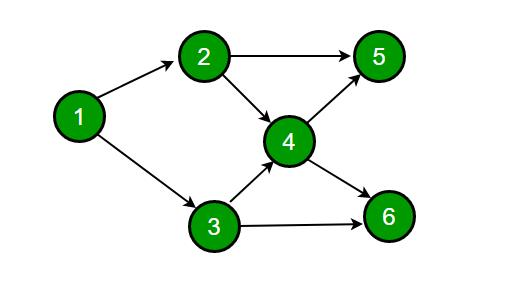Solution:

In option D, 1 appears after 2 and 3 which is not possible in Topological Sorting. In the given DAG it is directly visible that there is an outgoing edge from vertex 1 to vertex 2 and 3 hence 2 and 3 cannot come before vertex 1 so clearly option D is incorrect topological sort. But for questions in which it is not directly visible we should know how to find topological sort of a DAG.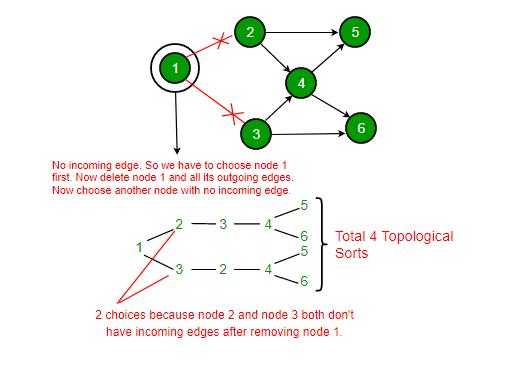QUESTION: 5

Let G be a weighted undirected graph and e be an edge with maximum weight in G. Suppose there is a minimum weight spanning tree in G containing the edge e. Which of the following statements is always TRUE?

Solution:

Background : Given a connected and undirected graph, a spanning tree of that graph is a subgraph that is a tree and connects all the vertices together.

• A spanning tree has exactly V - 1 edges.
• A single graph can have many different spanning trees. A minimum spanning tree (MST) or minimum weight spanning tree for a weighted, connected and undirected graph is a spanning tree with weight less than or equal to the weight of every other spanning tree. The weight of a spanning tree is the sum of weights given to each edge of the spanning tree.
• There can be more that one possible spanning trees of a graph. For example, the graph in this question has 6 possible spanning trees.
• MST has lightest edge of every cutset. Remember Prim's algorithm which basically picks the lightest edge from every cutset.

Choices of this question :

a) There exists a cutset in G having all edges of maximum weight : This is correct. If there is a heaviest edge in MST, then there exist a cut with all edges with weight equal to heavies edge. See point 4 discussed in above background.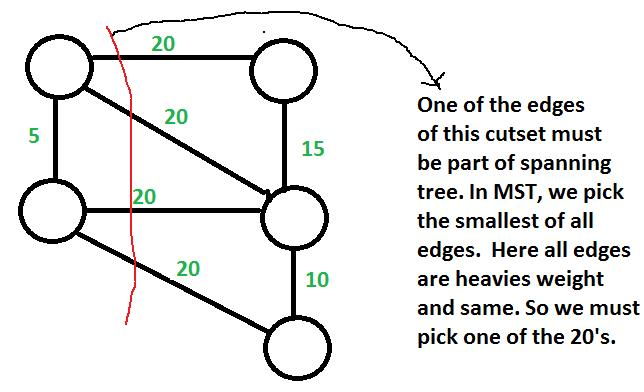b) There exists a cycle in G having all edges of maximum weight : Not always TRUE. The cutset of heaviest edge may contain only one edge. In fact there may be overall one edge of heavies weight which is part of MST (Consider a graph with two components which are connected by only one edge and this edge is the heavies)

c) Edge e cannot be contained in a cycle. Not Always True. The curset may form cycle with other edges. d) All edges in G have the same weight Not always True. As discussed in option b, there can be only one edge of heaviest weight.

QUESTION: 6

Let G be a graph with n vertices and m edges. What is the tightest upper bound on the running time on Depth First Search of G? Assume that the graph is represented using adjacency matrix.

Solution:

Depth First Search of a graph takes O(m+n) time when the graph is represented using adjacency list. In adjacency matrix representation, graph is represented as an "n x n" matrix. To do DFS, for every vertex, we traverse the row corresponding to that vertex to find all adjacent vertices (In adjacency list representation we traverse only the adjacent vertices of the vertex). Therefore time complexity becomes O(n2)

QUESTION: 7

The cyclomatic complexity of the flow graph of a program provides

Solution:

Option (C) is correct, because the cyclomatic complexity of the flow graph of a program provides an upper bound for the number of tests that must be conducted to ensure that all statements have been executed at least once.

QUESTION: 8

Consider the tree arcs of a BFS traversal from a source node W in an unweighted, connected, undirected graph. The tree T formed by the tree arcs is a data structure for computing.

Solution:

BFS always produces shortest path from source to all other vertices in an unweighted graph.

QUESTION: 9

What is the largest integer m such that every simple connected graph with n vertices and n edges contains at least m different spanning trees?

Solution:

A graph is connected iff all nodes can be traversed from each node. For a graph with n nodes, there will be n-1 minimum number of edges.
Given that there are n edges, that means a cycle is there in the graph.
The simplex graph with these conditions may be: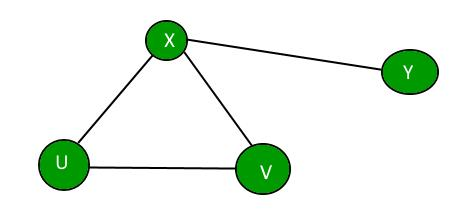Now we can make a different spanning tree by removing one edge from the cycle, one at a time.
Minimum cycle length can be 3, So, there must be atleast 3 spanning trees in any such Graph.

QUESTION: 10

Suppose depth first search is executed on the graph below starting at some unknown vertex. Assume that a recursive call to visit a vertex is made only after first checking that the vertex has not been visited earlier. Then the maximum possible recursion depth (including the initial call) is _________.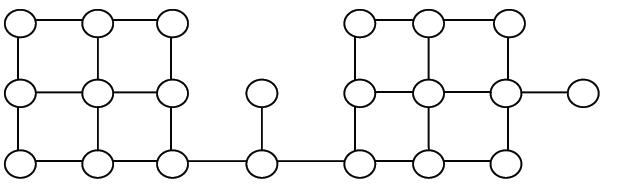Solution:

The following diagram shows the worst case situation where the recursion tree has maximum depth.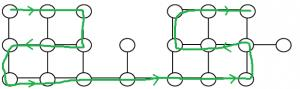So the recursion depth is 19 (including the first node).

QUESTION: 11

For the undirected, weighted graph given below, which of the following sequences of edges represents a correct execution of Prim's algorithm to construct a Minimum Span­ning Tree?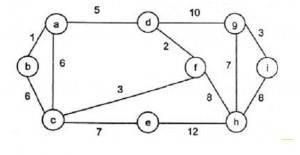Solution:

In prims algorithm we start with any node and keep on exploring minimum cost neighbors of nodes already covered.

QUESTION: 12

Let T be a depth first search tree in an undirected graph G. Vertices u and n are leaves of this tree T. The degrees of both u and n in G are at least 2. which one of the following statements is true?

Solution:

Below example shows that A and B are FALSE: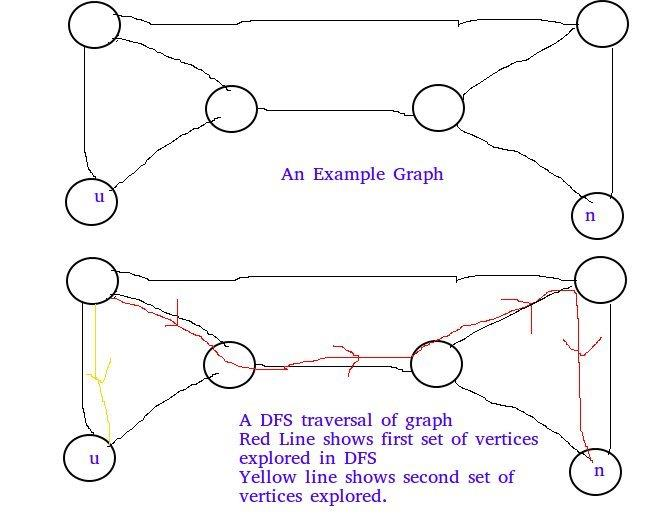Below example shows C is false: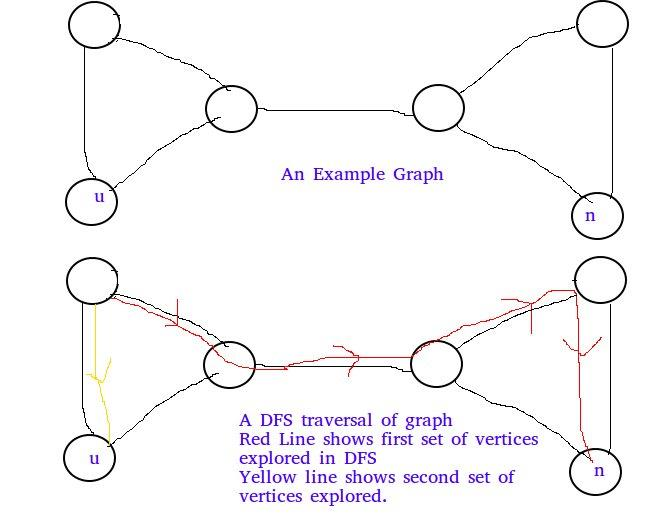QUESTION: 13

Consider a directed graph with n vertices and m edges such that all edges have same edge weights. Find the complexity of the best known algorithm to compute the minimum spanning tree of the graph?

Solution:

It’s a special case in which all edge weights are equal, so dfs would work and resultant depth first tree would be the spanning tree. So the answer would be O(m+n).

QUESTION: 14

Let G be an undirected graph. Consider a depth-first traversal of G, and let T be the resulting depth-first search tree. Let u be a vertex in G and let v be the first new (unvisited) vertex visited after visiting u in the traversal. Which of the following statements is always true?

Solution:

In DFS, if 'v' is visited
after 'u', then one of the following is true.
1) (u, v) is an edge.
u
/   \
v     w
/     / \
x     y   z

2) 'u' is a leaf node.
w
/   \
x     v
/     / \
u     y   z
In DFS, after visiting a node, we first recur for all unvisited children. If there are no unvisited children (u is leaf), then control goes back to parent and parent then visits next unvisited children.

QUESTION: 15

You are given a graph containing n vertices and m edges and given that the graph doesn’t contain cycle of odd length. Time Complexity of the best known algorithm to find out whether the graph is bipartite or not is ?

Solution:

It is by definition that a graph is bipartite iff it does not contain odd length cycles. So the answer is O(1).

QUESTION: 16

In a depth-first traversal of a graph G with n vertices, k edges are marked as tree edges. The number of connected components in G is

Solution:

Tree edges are the edges that are part of DFS tree.  If there are x tree edges in a tree, then  x+1 vertices in the tree. The output of DFS is a forest if the graph is disconnected.  Let us see below simple example where graph is disconnected.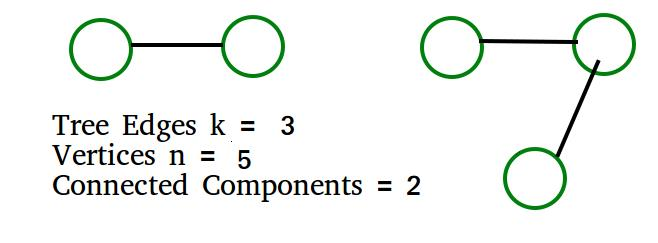The above example matches with D option More Examples:

1) All vertices  of Graph are connected.  k must be n-1.  We get number of connected components  = n- k =  n - (n-1) = 1

2) No vertex is connected. k must be 0.  We get number of connected components  = n- k =  n - 0 = n

QUESTION: 17

Let G be a simple graph with 20 vertices and 8 components. If we delete a vertex in G, then number of components in G should lie between ____.

Solution:

Case 1: If the vertex we are deleting from G is an isolated vertex, which is a component by itself, then number of components in G becomes 7.
Case 2: If G is a start Graph, then by deleting the cut vertex of G, we get 19 components.
Hence, number of components in G should lie between 7 and 19.

QUESTION: 18

Consider the following directed graph.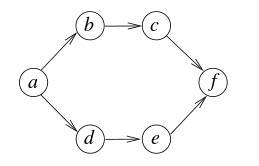The number of different topological orderings of the vertices of the graph is   Note : This question was asked as Numerical Answer Type.

Solution:

Following are different 6 Topological Sortings
a-b-c-d-e-f
a-d-e-b-c-f
a-b-d-c-e-f
a-d-b-c-e-f
a-b-d-e-c-f
a-d-b-e-c-f

QUESTION: 19

Let G be the graph with 100 vertices numbered 1 to 100. Two vertices i and j are adjacent iff |i−j|=8 or |i−j|=12. The number of connected components in G is

Solution:

When vertices are arranged with difference of 8 there are 8 components as shown by 8 columns in the image below: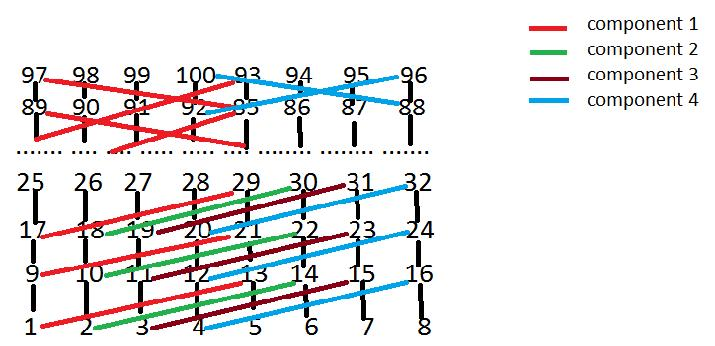When vertices are arranged withdifference of 12 the number of components is reduced to 4 as first column will be connected with fifth column, second column will be connected with sixth column, third column will be connected with seventh column and fourth column will be connected with eighth column. No other form of connection exists so total 4 connected components are there. So, option (B) is correct. This explanation is contributed by Pradeep Pandey.

QUESTION: 20

Let G = (V, G) be a weighted undirected graph and let T be a Minimum Spanning Tree (MST) of G maintained using adjacency lists. Suppose a new weighed edge (u, v) ∈ V×V is added to G. The worst case time complexity of determining if T is still an MST of the resultant graph is

Solution:
• As T is a minimum spanning tree and we need to add a new edge to existing spanning tree.
• Later we need to check still T is a minimum spanning tree or not, So we need to check all vertices whether there is any cycle present after adding a new edge.
• All vertices need to traverse to confirm minimum spanning tree after adding new edge then time complexity is O(V).

Option (D) is correct.